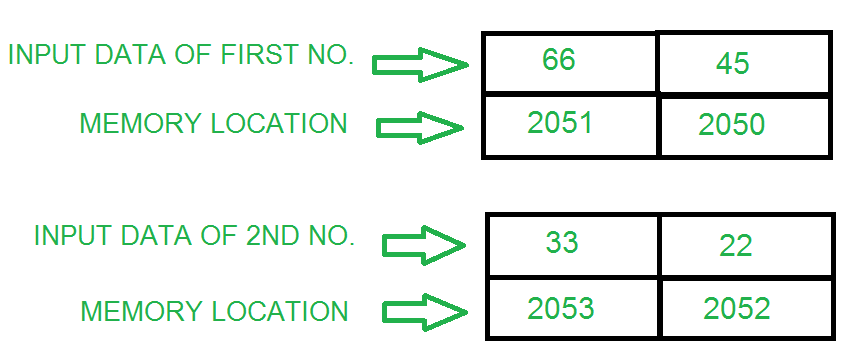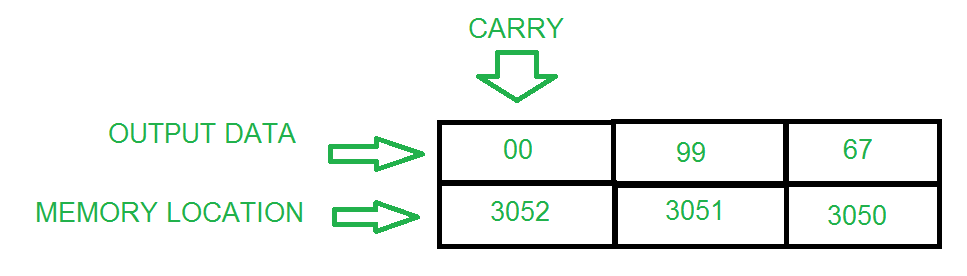Open in App
Not now

# 8085 program to add two 16 bit numbers

• Difficulty Level : Easy
• Last Updated : 07 Jul, 2022

Problem: Write an assembly language program to add two 16 bit numbers by using:

1. 8-bit operation
2. 16-bit operation

Example:###1. Addition of 16-bit numbers using 8-bit operation:

It is a lengthy method and requires more memory as compared to the 16-bit operation.

Algorithm:

1. Load the lower part of the first number in the B register.
2. Load the lower part of the second number in A (accumulator).
3. Add both the numbers and store.
4. Load the higher part of the first number in the B register.
5. Load the higher part of the second number in A (accumulator).
6. Add both the numbers with carrying from the lower bytes (if any) and store them at the next location.

Program:

Explanation:

1. LDA 2050 stores the value at 2050 in A (accumulator).
2. MOV B, A stores the value of A into the B register.
3. LDA 2052 stores the value at 2052 in A.
4. ADD B add the contents of B and A and store them in A.
5. STA 3050 stores the result in memory location 3050.
6. LDA 2051 stores the value at 2051 in A.
7. MOV B, A stores the value of A into the B register.
8. LDA 2053 stores the value at 2053 in A.
9. ADC B adds the contents of B, A, and carry from the lower bit addition and store in A.
10. STA 3051 stores the result in memory location 3051.
11. HLT stops execution.

### 2. Addition of 16 bit numbers using 16-bit operation:

It is a very short method and less memory is also required as compared to 8-bit operations.

Algorithm:

1. Load both the lower and the higher bits of the first number at once.
2. Copy the first number to another registered pair.
3. Load both the lower and the higher bits of second number at once.
4. Add both the register pairs and store the result in a memory location.

Program:

Explanation:

1. LHLD 2050 loads the value at 2050 in L register and that in 2051 in the H register (first number)
2. XCHG copies the content of the H to D register and L to E register
3. LHLD 2052 loads the value at 2052 in L register and that in 2053 in the H register (second number)
4. DAD D adds the value of H with D and L with E and stores the result in H and L
5. SHLD 3050 stores the result at memory location 3050
6. HLT stops execution
My Personal Notes arrow_drop_up